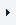LOGINREGISTER (FREE TRIAL)myTOMLAB
 OptimizationLinear ProgrammingQuadratic ProgrammingUnconstrained OptimizationConstrained Optimization, Nonlinear ProgrammingLinear Least SquaresConstrained Nonlinear Least SquaresBox-bounded Global OptimizationGlobal Optimization, costly functionsMixed-Integer ProgrammingMixed-Integer Quadratic ProgrammingMixed-Integer Nonlinear ProgrammingSemi-definite Programming

# Mixed-Integer Nonlinear Programming

 TOMLAB /KNITRO TOMLAB /MINLP

Tomlab /Knitro provides tools for solving optimization models (both linear and nonlinear) with binary or integer variables. Knitro offers three algorithms for mixed-integer nonlinear programming (MINLP). The first is a nonlinear branch and bound method, the second implements the hybrid Quesada-Grossman method for convex MINLP, and the third implements a mixed-integer Sequential Quadratic Programming (MISQP) method that is able to handle non-relaxable integer variables. Knitro is designed for convex mixed integer programming and is only a heuristic for nonconvex problems. The MINLP code also handles mixed integer linear programs (MILP) of moderate size.

If the user is able to provide at least first derivatives for both the objective and the nonlinear constraints, or automatic differentiation using TOMLAB /MAD is possible to apply to obtain first derivatives, the minlpBB solver in TOMLAB /MINLP is a good choice. minlpBB is using an outer approximation branch- and bound algorithm and solves problems that are convex in the continuous variables, but also many non-convex problems. The result for minlpBB on non-convex problems is dependent on the initial guess of the unknowns. Another approach for MINLP is the TOMLAB /OQNLP solver, that combines statistical sampling methods with intelligent choices of initial points for local searches, hopefully finding the global minimum among the local minima found.

For low-dimensional black-box problems, the glcCluster solver in the TOMLAB Base Module offers a similar solution as TOMLAB /OQNLP. Here the sampling is deterministic by applying the DIRECT algorithm as implemented in the glcDirect (or glcSolve, glcFast) solver. A clustering algorithm is then applied on the sampled points to find a good set of initial points to do local searches for. By default glcCluster is using conSolve for the local searches, but any TOMLAB nonlinear programming solver could be used, and recommended is NPSOL, DNOPT or SNOPT in TOMLAB /SOL.

Another approach for low-dimensional black-box problems is to run glcDirect for a larger number of iterations, if the needed accuracy is not that high. This is especially appropriate is the function is noisy or non-smooth, and derivative based methods are likely to have trouble.

If the problems are time-consuming (costly, CPU-intensive) another option is to solve the problems using one of the global solvers included in TOMLAB /CGO, such as rbfSolve or EGO.

Solver reference:

 TOMLAB /MINLP - minlpBB TOMLAB Base Module - glcCluster optionally with e.g. TOMLAB /NPSOL TOMLAB /KNITRO - KNITRO TOMLAB Base Module - glcDirect TOMLAB Base Module - glcSolve